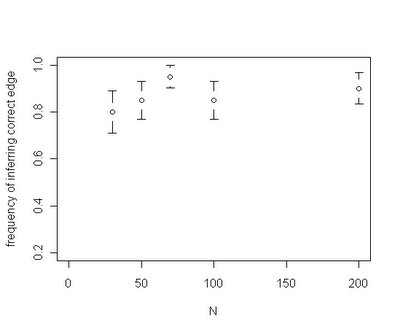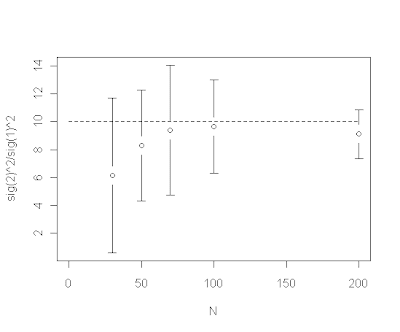## Tuesday, May 10, 2011

### Parameter estimates from evol.rate.mcmc()

My coauthors (1,2,3) and I are nearing completion of the revisions for the manuscript describing our MCMC method for identifying the location of a shift in the evolutionary rate through time. I have described the approach and our methods for collapsing the posterior sample in several prior posts (e.g., 1,2,3,4,5,6,7).

Among the interesting results that have been revealed so far - for a given rate shift our capacity to identify the correct edge on the tree where the rate shift occurred seems to be fairly insensitive to the total size of the tree. For instance, the plot below is for trees of size ranging from N=30 through N=200:However, we have also found that the ratio of estimated rates (i.e., σ2212) is downwardly biased for small trees, e.g., from an example in which the generating rate ratio σ2212=10:In other words, for small trees the estimated evolutionary rates are too similar relative to the generating rates. We believe, and explain in our manuscript, that this is a direct consequence of integrating across uncertainty in the location of the rate shift (thanks to BR for this insight). For a given rate shift, the posterior density for the location of the shift tends to be much more diffuse on a smaller tree. It makes sense therefore that the estimated difference in evolutionary rates (here, the ratio) will be downwardly biased because we have many posterior samples on incorrect branches where (at least on one side of this split) low and high rates are averaged.

By the way, the plots above were created using plotCI() from the {gplots} package.